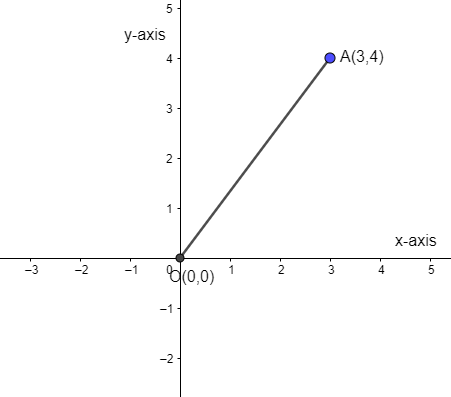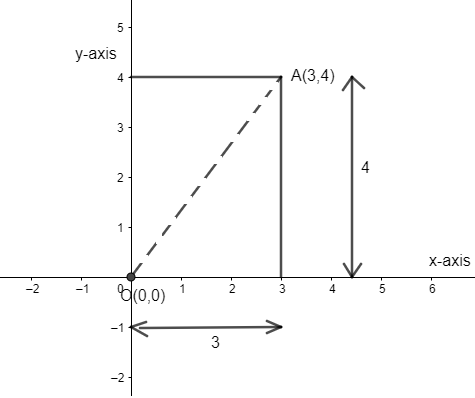QUESTION

# Find the distance from origin to the point $\left( 3,4 \right)$.

Hint: Origin is a point of intersection of coordinate axes and coordinates of origin are given as $\left( 0,0 \right)$. Now, find the distance between origin and the given point in the problem using the distance formula, used for calculating distance between two points. It is given as
$\sqrt{{{\left( {{x}_{1}}-{{x}_{2}} \right)}^{2}}+{{\left( {{y}_{1}}-{{y}_{2}} \right)}^{2}}}$
Where coordinated of two points are $\left( {{x}_{1}},{{y}_{1}} \right)$ and $\left( {{x}_{2}},{{y}_{2}} \right)$.

As we know the origin is a point where the axes of the cartesian system intersect each other, other points can then be located with the reference to the origin by giving their numerical coordinates that is the position of their projections along each axis. And the coordinates of origin are given as $\left( 0,0 \right)$.

Now, we have to determine the distance of the point whose coordinates are $\left( 3,4 \right)$ from the origin $\left( 0,0 \right)$. So, we can represent point $\left( 3,4 \right)$ to the coordinate axes asAs we know the distance between any two points on the 2-dimensional (x axis and y axis) plane can be given using the distance formula. We can give the distance between the points $P\left( {{x}_{1}},{{y}_{1}} \right)$ and $Q\left( {{x}_{2}},{{y}_{2}} \right)$ by the distance formula as

PQ $=\sqrt{{{\left( {{x}_{1}}-{{x}_{2}} \right)}^{2}}+{{\left( {{y}_{1}}-{{y}_{2}} \right)}^{2}}}$ ………………………………………(i)

So, we have two points $\left( 3,4 \right)$ and $\left( 0,0 \right)$, where we need to determine the distance between them. So, we can take point $\left( 3,4 \right)$ as $A\left( 3,4 \right)$ and point $\left( 0,0 \right)$ as $O\left( 0,0 \right)$. We can put the values of the coordinates to the expression given in equation (i) to get the distance between both the points i.e. OA.

So, put $\left( {{x}_{1}},{{y}_{1}} \right)=\left( 0,0 \right)$ and $\left( {{x}_{2}},{{y}_{2}} \right)=\left( 3,4 \right)$

$\Rightarrow {{x}_{1}}=0,{{y}_{1}}=0$ and ${{x}_{2}}=3,{{y}_{2}}=4$.

Hence, we get distance OA as

OA $=\sqrt{{{\left( 0-3 \right)}^{2}}+{{\left( 0-4 \right)}^{2}}}$

OA $=\sqrt{{{\left( -3 \right)}^{2}}+{{\left( -4 \right)}^{2}}}=\sqrt{9+16}$

OA $=\sqrt{25}=5$

Where squares of the numbers ‘-3’ ad ‘-4’ will be ‘9’ and ‘16’ respectively. And we know the square root of 25 is 5.

Hence, the distance OA or distance between origin and $\left( 3,4 \right)$ is 5.

So, ‘5’ is the correct answer to the given problem.

Note: Another approach for the question would be that we can use Pythagoras theorem to get the distance between origin and $\left( 3,4 \right)$ in following way :-